Courses

# RD Sharma Solutions - Ex-19.1 & 19.2, Visualising Solid Shapes, Class 7, Math Class 7 Notes | EduRev

## Class 7 : RD Sharma Solutions - Ex-19.1 & 19.2, Visualising Solid Shapes, Class 7, Math Class 7 Notes | EduRev

The document RD Sharma Solutions - Ex-19.1 & 19.2, Visualising Solid Shapes, Class 7, Math Class 7 Notes | EduRev is a part of the Class 7 Course RD Sharma Solutions for Class 7 Mathematics.
All you need of Class 7 at this link: Class 7

#### Question 1:

Complete the following table and verify Euler's formula in each case.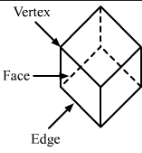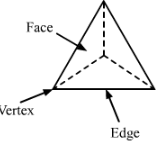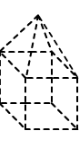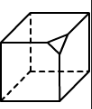Faces (F) 6 4 9 7 Edges (E) 12 6 16 15 Vertex (V) 8 4 9 10

 I II III IV Faces (F) 6 4 9 7 Edges (E) 12 6 16 15 Vertices (V) 8 4 9 10 Euler's formula (F − E + V) 6 − 12 + 8 = 2 4 − 6 + 4 = 2 9 − 16 + 9 = 2 7 − 15 + 10 = 2

Hence Euler's formula is verified for these figures.

#### Question 2:

Give three examples from our daily life which are in the form of (i) a cone (ii) a sphere (iii) a cuboid (iv) a cylinder (v) a pyramid.

Exercise 19.2

#### Question 1:

Match the following nets with appropriate solids: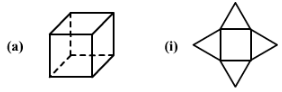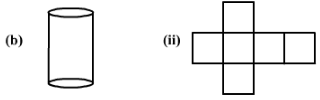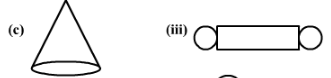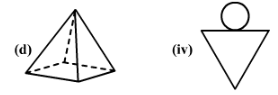Here
(a) → (ii)
(b) → (iii)
(c) → (iv)
(d) → (i)

#### Question 2:

Identify the nets which can be used to make cubes (cut-out the nets and try it):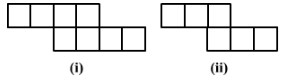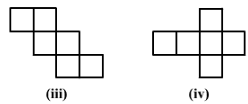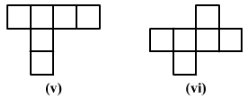Only (ii), (iv) and (vi) form a cube.

#### Question 3:

Can the following be a net for a die? Explain your answer.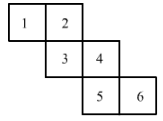Since, in a die, the sum of the number of opposite faces of a die is 7. In the given figure, it is not possible to get the sum as 7. Hence the given net is not suitable for a die.

#### Question 4:

Out of the following four nets there are two correct nets to make a tetrahedron. Identify them.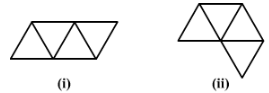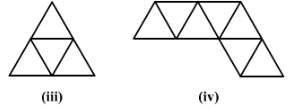For making a tetrahedron, only (i) and (iii) are suitable nets.

#### Question 5:

Here is an incomplete net for making a cube. Complete it in atleast two different ways.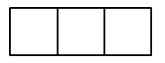The complete nets for making a cube are Images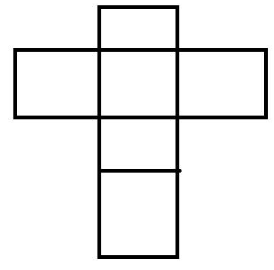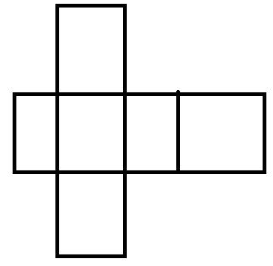Offer running on EduRev: Apply code STAYHOME200 to get INR 200 off on our premium plan EduRev Infinity!

97 docs

,

,

,

,

,

,

,

,

,

,

,

,

,

,

,

,

,

,

,

,

,

,

,

,

,

,

,

,

,

,

;